# Python Join List

Published on August 3, 2022By Pankaj
Developer and author at DigitalOcean.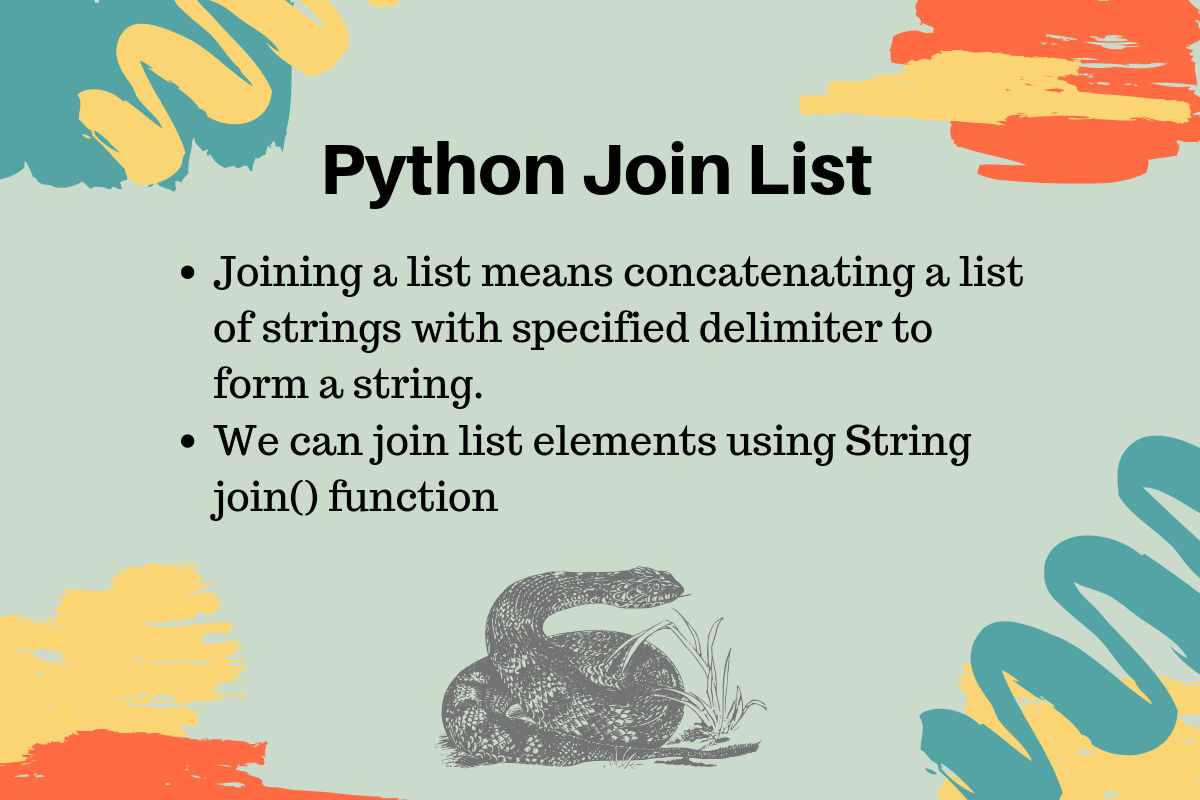While we believe that this content benefits our community, we have not yet thoroughly reviewed it. If you have any suggestions for improvements, please let us know by clicking the “report an issue“ button at the bottom of the tutorial.

Python join list means concatenating a list of strings with a specified delimiter to form a string. Sometimes it’s useful when you have to convert list to string. For example, convert a list of alphabets to a comma-separated string to save in a file.

## Python Join List

We can use python string join() function to join a list of strings. This function takes `iterable` as argument and List is an interable, so we can use it with List. Also, the list should contain strings, if you will try to join a list of ints then you will get an error message as `TypeError: sequence item 0: expected str instance, int found`. Let’s look at a short example for joining list in python to create a string.

``````vowels = ["a", "e", "i", "o", "u"]

vowelsCSV = ",".join(vowels)

print("Vowels are = ", vowelsCSV)
``````

When we run the above program, it will produce the following output.

``````Vowels are =  a,e,i,o,u
``````

### Python join two strings

We can use join() function to join two strings too.

``````message = "Hello ".join("World")

print(message) #prints 'Hello World'
``````

### Why join() function is in String and not in List?

One question arises with many python developers is why the join() function is part of String and not list. Wouldn’t below syntax be more easy to remember and use?

``````vowelsCSV = vowels.join(",")
``````

There is a popular StackOverflow question around this, here I am listing the most important points from the discussions that makes total sense to me.

The main reason is that join() function can be used with any iterable and result is always a String, so it makes sense to have this function in String API rather than having it in all the iterable classes.

### Joining list of multiple data-types

Let’s look at a program where we will try to join list items having multiple data types.

``````names = ['Java', 'Python', 1]
delimiter = ','
single_str = delimiter.join(names)
print('String: {0}'.format(single_str))
``````

Let’s see the output for this program: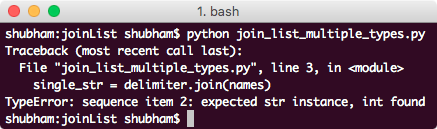This was just a demonstration that a list which contains multiple data-types cannot be combined into a single String with `join()` function. List must contain only the String values.

### Split String using join function

We can use `join()` function to split a string with specified delimiter too.

``````names = 'Python'
delimiter = ','
single_str = delimiter.join(names)
print('String: {0}'.format(single_str))
``````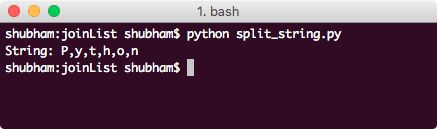This shows that when String is passed as an argument to join() function, it splits it by character and with the specified delimiter.

### Using split() function

Apart from splitting with the `join()` function, `split()` function can be used to split a String as well which works almost the same way as the `join()` function. Let’s look at a code snippet:

``````names = ['Java', 'Python', 'Go']
delimiter = ','
single_str = delimiter.join(names)
print('String: {0}'.format(single_str))

split = single_str.split(delimiter)
print('List: {0}'.format(split))
``````

Let’s see the output for this program: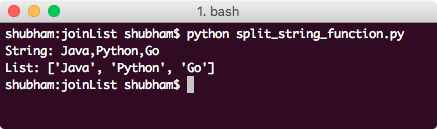We used the same delimiter to split the String again to back to the original list.

### Splitting only n times

The `split()` function we demonstrated in the last example also takes an optional second argument which signifies the number of times the splot operation should be performed. Here is a sample program to demonstrate its usage:

``````names = ['Java', 'Python', 'Go']
delimiter = ','
single_str = delimiter.join(names)
print('String: {0}'.format(single_str))

split = single_str.split(delimiter, 1)
print('List: {0}'.format(split))
``````

Let’s see the output for this program: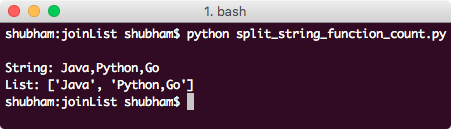This time, split operation was performed only one time as we provided in the `split()` function parameter. That’s all for joining a list to create a string in python and using split() function to get the original list again.Pankaj

author

Developer and author at DigitalOcean.

#### Still looking for an answer?

message = "Hello ".join(“World”) print(message) #prints ‘Hello World’ This actually prints: ‘WHello oHello rHello lHello d’

- Miguel